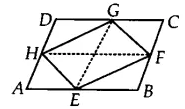Q

# If E,F,G and H are respectively the mid-points of the sides of a parallelogram ABCD, show that ar (EFGH) = 1/2 ar (ABCD)

Q: 2     If  E, F, G and H are respectively the mid-points of the sides of a parallelogram ABCD, show that

Views

Join GE and HE,
Since F, F, G, H are the mid-points of the parallelogram ABCD. Therefore, GE || BC ||AD and HF || DC || AB.
It is known that if a triangle and the parallelogram are on the same base and between the same parallel lines. then the area of the triangle is equal to the half of the area of the parallelogram.Now, EFG and ||gm BEGC are on the same base and between the same parallels EG and BC.
Therefore, ar (EFG) =  ar (||gm BEGC)...............(i)
Similarly, ar (EHG) = 1/2 . ar(||gm AEGD)..................(ii)
By adding eq (i) and eq (ii), we get

ar (EFG) +  ar (EHG) = 1/2 (ar (||gm BEGC) + ar(||gm AEGD))
ar (EFGH) = 1/2 ar(ABCD)
Hence proved

Exams
Articles
Questions